## RS Aggarwal Class 9 Solutions Chapter 15 Probability Ex 15A

These Solutions are part of RS Aggarwal Solutions Class 9. Here we have given RS Aggarwal Class 9 Solutions Chapter 15 Probability Ex 15A.

Question 1.
Solution:
Number of trials = 500 times
Let E be the no. of events in each case, then
∴No. of heads (E1) = 285 times
and no. of tails (E2) = 215 times
∴ Probability in each case will be
∴(i)P(E1) = $$\frac { 285 }{ 500 }$$ = $$\frac { 57 }{ 100 }$$ = 0.57
(ii) P(E2) = $$\frac { 215 }{ 500 }$$ = $$\frac { 43 }{ 100 }$$ = 0.43

Question 2.
Solution:
No. of trials = 400
Let E be the no. of events in each case, then
No. of 2 heads (E1) = 112
No. of one head (E2) = 160 times
and no. of O. head (E3) = 128 times
∴ Probability in each case will be:
∴ (i)P(E1) = $$\frac { 112 }{ 400 }$$ = $$\frac { 28 }{ 100 }$$ = 0.28
(ii)P(E2) = $$\frac { 160 }{ 400 }$$ = $$\frac { 40 }{ 100 }$$= 0.40
(iii) P(E3) = $$\frac { 128 }{ 400 }$$ = $$\frac { 32 }{ 100 }$$ = 0.32 Ans.

Question 3.
Solution:
Number of total trials = 200
Let E be the no. of events in each case, then
No. of three heads (E1) = 39 times
No. of two heads (E2) = 58 times
No. of one head (E3) = 67 times
and no. of no head (E4) = 36 times
∴ Probability in each case will be .
(i) P(E1) = $$\frac { 39 }{ 200 }$$ = 0.195
(ii) P(E3) = $$\frac { 67 }{ 200 }$$ = 0.335
(iii) P(E4) = $$\frac { 36 }{ 200 }$$ = $$\frac { 18 }{ 100 }$$ = 0.18
(iv) P(E2) = $$\frac { 58 }{ 200 }$$ = $$\frac { 29 }{ 100 }$$ = 0.29

Question 4.
Solution:
Solution No. of trials = 300 times
Let E be the no. of events in each case, then
No. of outcome of 1(E1) = 60
No. of outcome of 2(E2) = 72
No. of outcome of 3(E3) = 54
No. of outcome of 4(E4) 42
No. of outcome of 5(E5) = 39
No. of outcome of 6(E6) = 33
The probability of
(i) P(E3) = $$\frac { 54 }{ 300 }$$ = $$\frac { 18 }{ 100 }$$ = 0.18
(ii) P(E6) = $$\frac { 33 }{ 100 }$$ = $$\frac { 11 }{ 100 }$$= 0.11
(iii) P(E5) = $$\frac { 39 }{ 300 }$$ = $$\frac { 13 }{ 100 }$$ = 0.13
(iv) P(E1) = $$\frac { 60 }{ 300 }$$ = $$\frac { 20 }{ 100 }$$= 0.20 Ans.

Question 5.
Solution:
Let E be the no. of events in each case.
No. of ladies who like coffee (E1) = 142
No. of ladies who like coffee (E2) = 58
Probability of
(1) P(E1) = $$\frac { 142 }{ 200 }$$ = $$\frac { 71 }{ 100 }$$ = 0.71
(ii) P(E2) = $$\frac { 58 }{ 200 }$$ = $$\frac { 29 }{ 100 }$$ = 0.29 Ans.

Question 6.
Solution:
Total number of tests = 6
No. of test in which the students get more than 60% mark = 2
Probability will he
P(E) = $$\frac { 2 }{ 6 }$$ = $$\frac { 1 }{ 3 }$$Ans.

Question 7.
Solution:
No. of vehicles of various types = 240
No. of vehicles of two wheelers = 64.
Probability will be P(E) = $$\frac { 84 }{ 240 }$$ = $$\frac { 7 }{ 20 }$$ = 0.35 Ans.

Question 8.
Solution:
No. of phone numbers are one page = 200
Let E be the number of events in each case,
Then (i) P(E5) = $$\frac { 24 }{ 200 }$$ = $$\frac { 12 }{ 100 }$$ = 0.12
(ii) P(E8) = $$\frac { 16 }{ 200 }$$ = $$\frac { 8 }{ 100 }$$ = 0.08 Ans.

Question 9.
Solution:
No. of students whose blood group is checked = 40
Let E be the no. of events in each case,
Then (i) P(E0) = $$\frac { 14 }{ 40 }$$ = $$\frac { 7 }{ 20 }$$ = 0.35
(ii) P(EAB) = $$\frac { 6 }{ 40 }$$ = $$\frac { 3 }{ 20 }$$ = 0.15 Ans.

Question 10.
Solution:
No. of total students = 30.
Let E be the number of elements, this probability will be of interval 21 – 30
P(E) = $$\frac { 6 }{ 30 }$$ = $$\frac { 1 }{ 5 }$$ = 0.2 Ans.

Question 11.
Solution:
Total number of patients of various age group getting medical treatment = 360
Let E be the number of events, then
(i) No. of patient which are 30 years or more but less than 40 years = 60.
P(E) = $$\frac { 60 }{ 360 }$$ = $$\frac { 1 }{ 6 }$$
(ii) 50 years or more but less than 70 years = 50 + 30 = 80
P(E) = $$\frac { 80 }{ 360 }$$ = $$\frac { 2 }{ 9 }$$
(iii) Less than 10 years = zero
P(E) = $$\frac { 0 }{ 360 }$$ = 0
(iv) 10 years or more 90 + 50 + 60 + 80 + 50 + 30 = 360

Hope given RS Aggarwal Class 9 Solutions Chapter 15 Probability Ex 15A are helpful to complete your math homework.

If you have any doubts, please comment below. Learn Insta try to provide online math tutoring for you.

## RS Aggarwal Class 9 Solutions Chapter 14 Statistics Ex 14F

These Solutions are part of RS Aggarwal Solutions Class 9. Here we have given RS Aggarwal Solutions Class 9 Chapter 14 Statistics Ex 14F.

Other Exercises

Question 1.
Solution: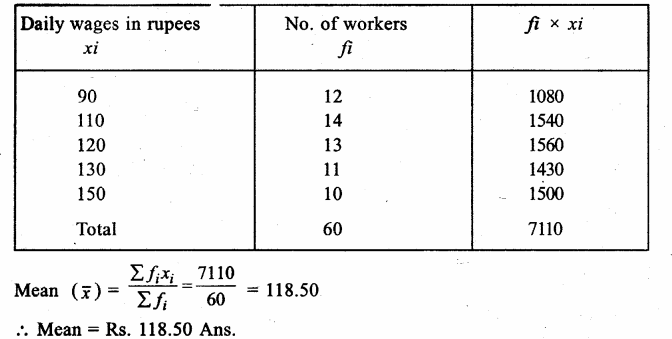Question 2.
Solution: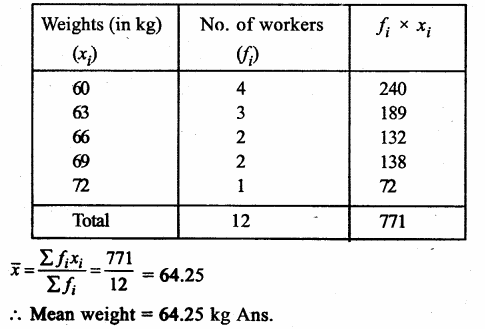Question 3.
Solution: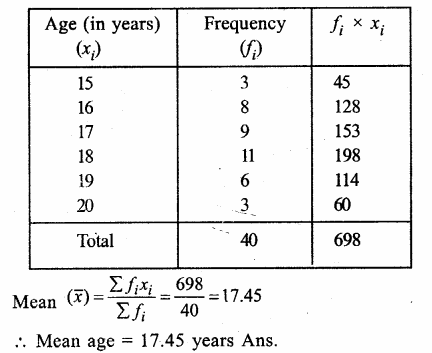Question 4.
Solution: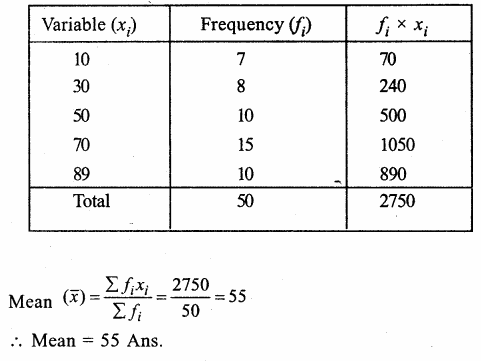Question 5.
Solution:
Mean = 8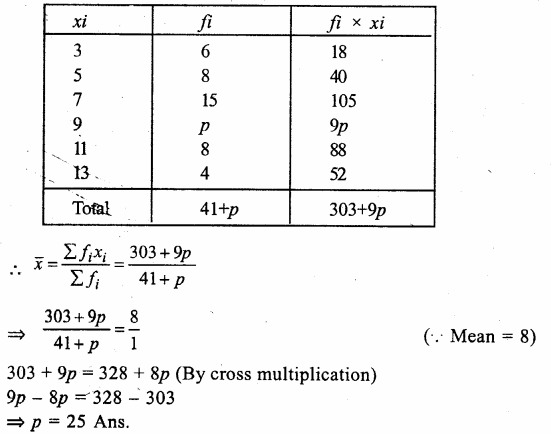Question 6.
Solution:
Mean = 28.25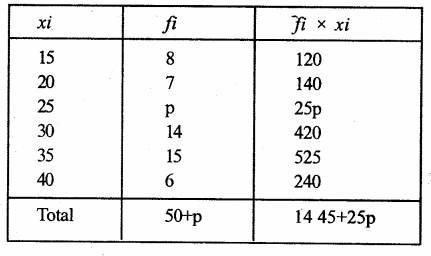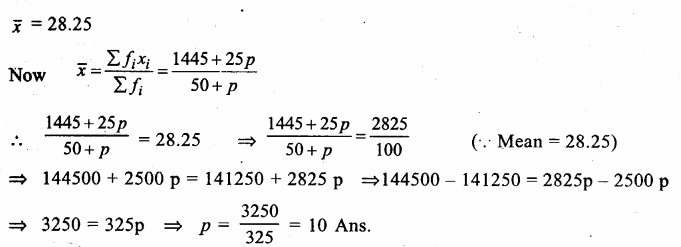Question 7.
Solution:
Mean = 16.6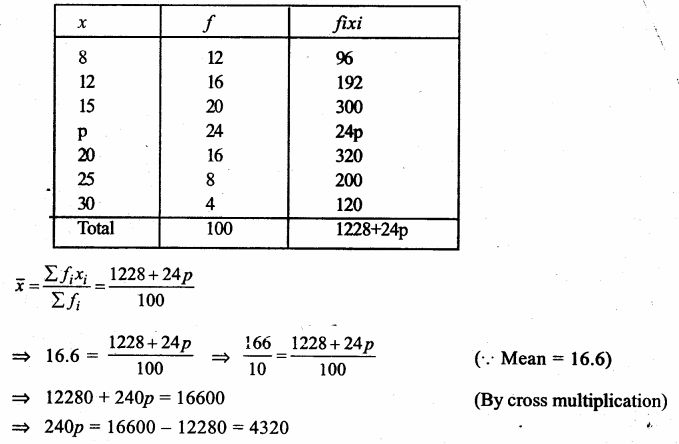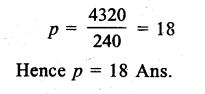Question 8.
Solution:
Mean = 50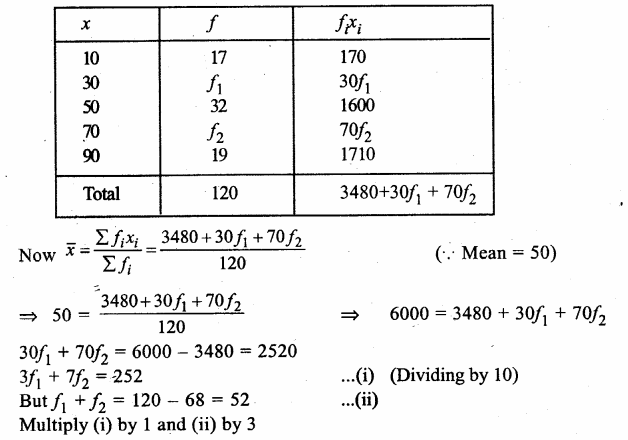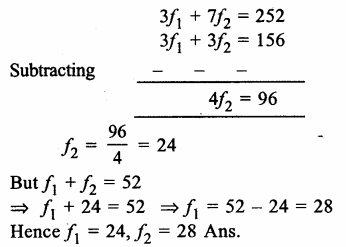Question 9.
Solution: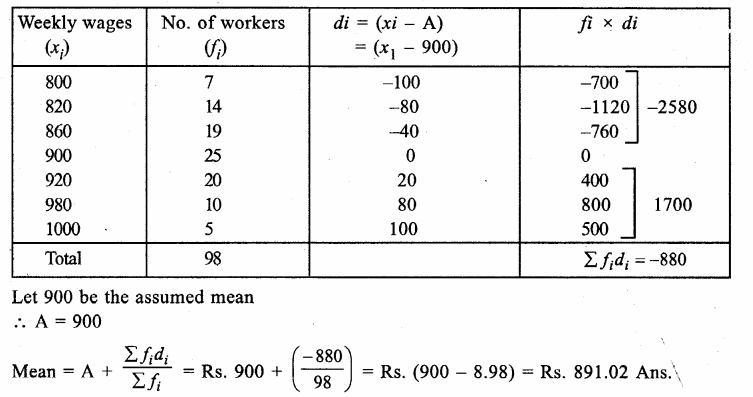Question 10.
Solution:
Let assumed mean = 67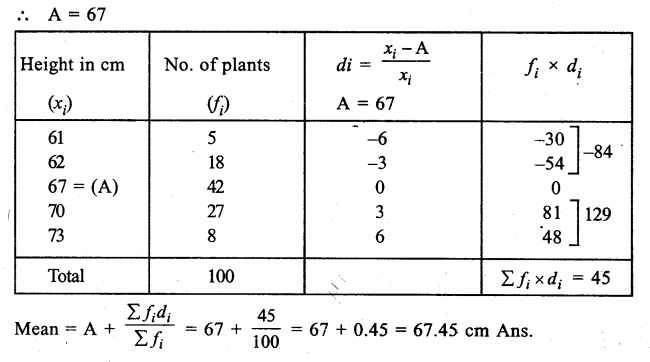Question 11.
Solution:
Here h = 1, Let assumed mean (A) = 21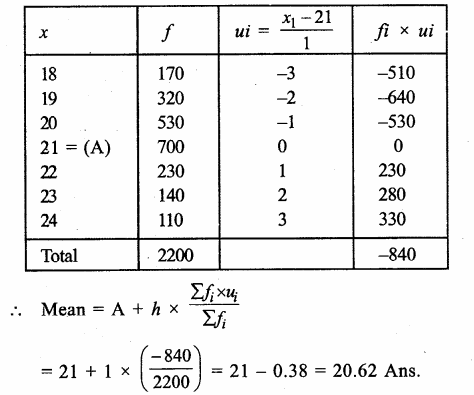Question 12.
Solution:
Here h = 400 and let assumed mean (A) = 1000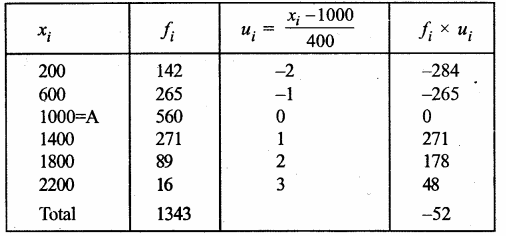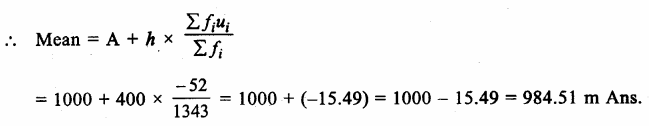Hope given RS Aggarwal Solutions Class 9 Chapter 14 Statistics Ex 14F are helpful to complete your math homework.

If you have any doubts, please comment below. Learn Insta try to provide online math tutoring for you.

## RS Aggarwal Class 9 Solutions Chapter 14 Statistics Ex 14H

These Solutions are part of RS Aggarwal Solutions Class 9. Here we have given RS Aggarwal Solutions Class 9 Chapter 14 Statistics Ex 14H.

Other Exercises

Question 1.
Solution:
Arranging the given data in ascending order :
0, 0, 1, 2, 3, 4, 5, 5, 6, 6, 6, 6
We see that 6 occurs in maximum times.
Mode = 6 Ans.

Question 2.
Solution:
Arranging in ascending order, we get:
15, 20, 22, 23, 25, 25, 25, 27, 40
We see that 25 occurs in maximum times.
Mode = 25 Ans.

Question 3.
Solution:
Arranging in ascending order we get:
1, 1, 2, 3, 3, 4, 5, 5, 6, 6, 7, 8, 9, 9, 9, 9, 9
Here, we see that 9 occurs in maximum times.
Mode = 9 Ans.

Question 4.
Solution:
Arranging in ascending order, we get:
9, 19, 27, 28, 30, 32, 35, 50, 50, 50, 50, 60
Here, we see that 50 occurs in maximum times.
Modal score = 50 scores Ans.

Question 5.
Solution:
Arranging in ascending order, we get:
10, 10, 11, 11, 12, 12, 13, 14,15, 17
Here, number of terms is 10, which is even
∴ Median = $$\frac { 1 }{ 2 } \left[ \frac { 10 }{ 2 } th\quad term+\left( \frac { 10 }{ 2 } +1 \right) th\quad term \right]$$
= $$\frac { 1 }{ 2 }$$ (5th term + 6th term)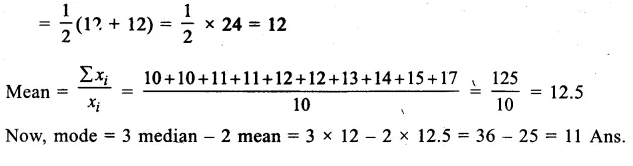Question 6.
Solution: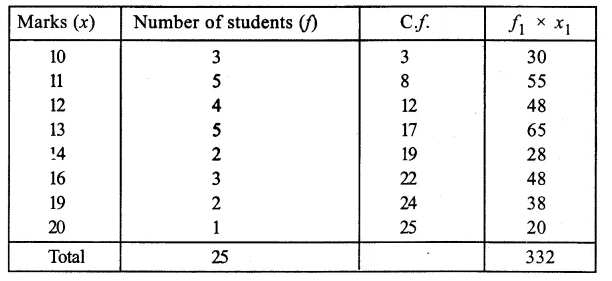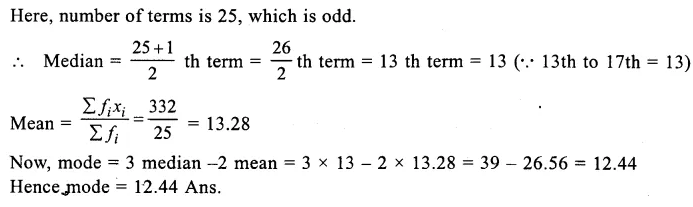Question 7.
Solution:
Writing its cumulative frequency table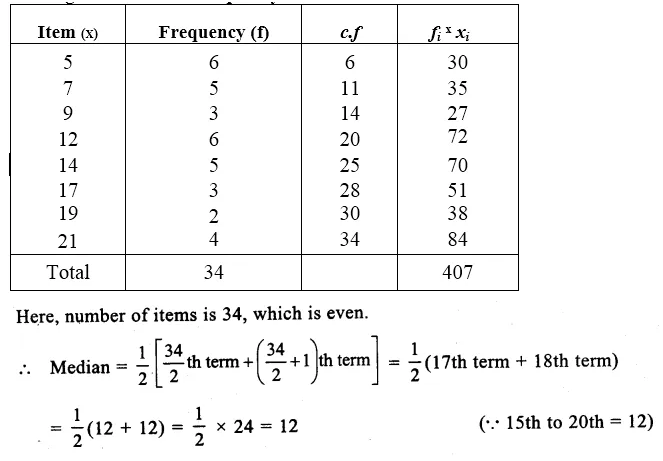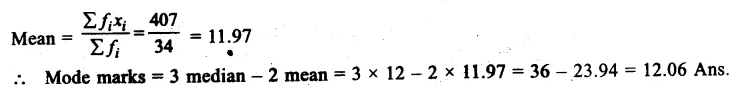Question 8.
Solution:
Writing its cumulative frequency table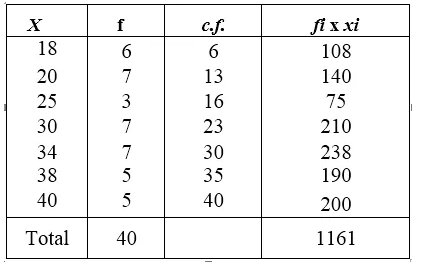Here, number of items is 40 which is even.
∴ Median = $$\frac { 1 }{ 2 } \left[ \frac { 40 }{ 2 } th\quad term+\left( \frac { 40 }{ 2 } +1 \right) th\quad term \right]$$
= $$\frac { 1 }{ 2 }$$ (20th term + 21th term)
= $$\frac { 1 }{ 2 }$$ (30 + 30) = $$\frac { 1 }{ 2 }$$ x 60 = 30
Mean= $$\frac { \sum { { f }_{ i }{ x }_{ i } } }{ \sum { { f }_{ i } } }$$ = $$\frac { 1161 }{ 40 }$$ = 29.025
∴Mode = 3 median – 2 mean = 3 x 30 – 2 x 29.025 = 90 – 58.05 = 31.95

Question 9.
Solution:
Preparing its cumulative frequency table we get: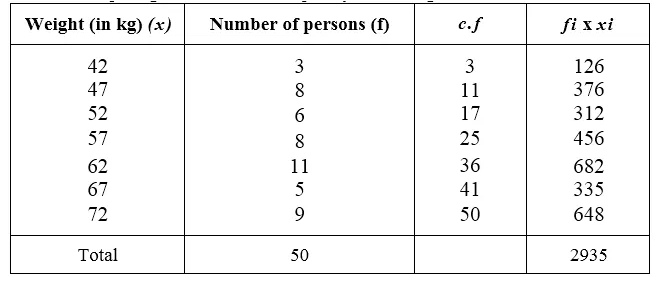Here number of terms is 50, which is even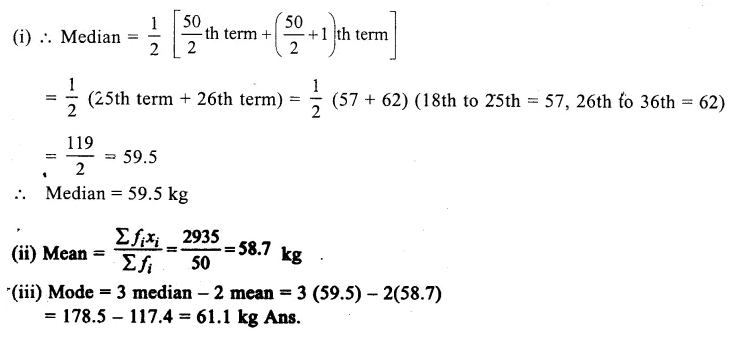Question 10.
Solution:
Preparing its cumulative frequency table :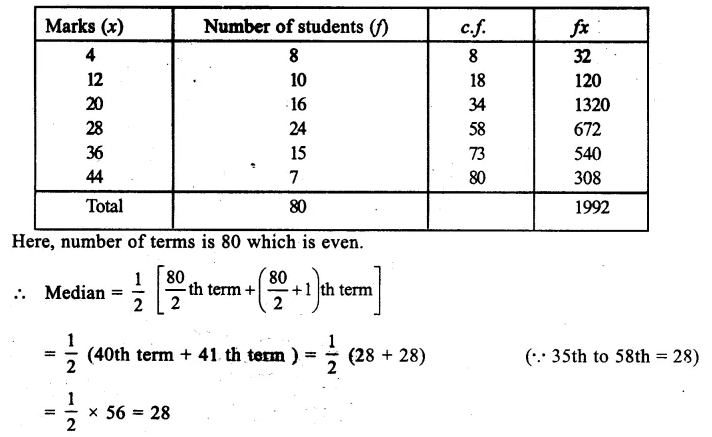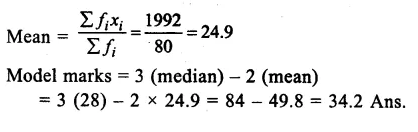Question 11.
Solution:
Preparing its cumulative frequency table we have,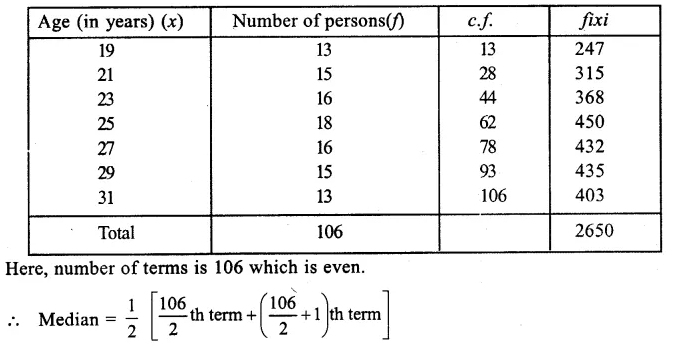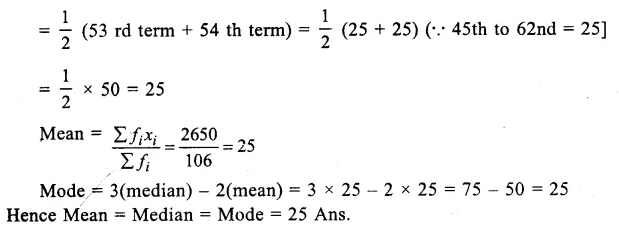Question 12.
Solution:
Preparing its cumulative frequency table we have,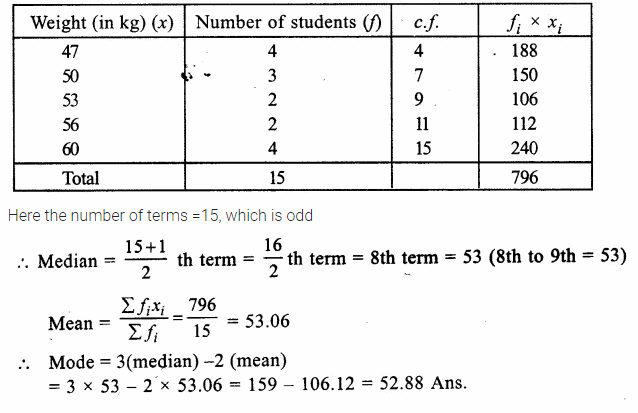Hope given RS Aggarwal Solutions Class 9 Chapter 14 Statistics Ex 14H are helpful to complete your math homework.

If you have any doubts, please comment below. Learn Insta try to provide online math tutoring for you.

## RS Aggarwal Class 9 Solutions Chapter 14 Statistics Ex 14G

These Solutions are part of RS Aggarwal Solutions Class 9. Here we have given RS Aggarwal Solutions Class 9 Chapter 14 Statistics Ex 14G.

Other Exercises

Question 1.
Solution:
Arranging in ascending order, we get:
2,2,3,5,7,9,9,10,11
Here, number of terms is 9 which is odd.
∴ Median = $$\frac { n+1 }{ 2 }$$ th term = $$\frac { 9+1 }{ 2 }$$ th term = 5th term = 7 Ans.
(ii) Arranging in ascending order, we get: 6, 8, 9, 15, 16, 18, 21, 22, 25
Here, number of terms is 9 which is odd.
∴ Median = $$\frac { n+1 }{ 2 }$$ th term = $$\frac { 9+1 }{ 2 }$$ th term = 5th term = 16 Ans.
(iii) Arranging in ascending order, we get: 6, 8, 9, 13, 15, 16, 18, 20, 21, 22, 25
Here, number of terms is 11 which is odd.
∴ Median = $$\frac { 11+1 }{ 2 }$$ th term = $$\frac { 12 }{ 2 }$$ th term = 6th term = 16 Ans.
(iv) Arranging in ascending order, we get:
0, 1, 2, 2, 3, 4, 4, 5, 5, 7, 8, 9, 10
Here, number of terms is 13, which is odd.
Median = $$\frac { 13+1 }{ 2 }$$ th term = $$\frac { 14 }{ 2 }$$ th term = 7th term = 4 Ans.

Question 2.
Solution:
Arranging in ascending order, we get 9, 10, 17, 19, 21, 22, 32, 35
Here, number of terms is 8 which is even
∴Median = $$\frac { 1 }{ 2 } \left[ \frac { 8 }{ 2 } th\quad term+\left( \frac { 8 }{ 2 } +1 \right) th\quad term \right]$$
= $$\frac { 1 }{ 2 }$$ [4th term + 5th term] = $$\frac { 1 }{ 2 }$$ (19 + 21) = $$\frac { 1 }{ 2 }$$ x 40 = 20
(ii) Arranging in ascending order, we get:
29, 35, 51, 55, 60, 63, 72, 82, 85, 91
Here number of terms is 10 which is even
∴Median = $$\frac { 1 }{ 2 } \left[ \frac { 10 }{ 2 } th\quad term+\left( \frac { 10 }{ 2 } +1 \right) th\quad term \right]$$
= $$\frac { 1 }{ 2 }$$ (60 + 63) = $$\frac { 1 }{ 2 }$$ x 123 = 61.5 Ans.
(iii) Arranging in ascending order we get
3, 4, 9, 10, 12, 15, 17, 27, 47, 48, 75, 81
Here number of terms is 12 which is even.
∴Median = $$\frac { 1 }{ 2 } \left[ \frac { 12 }{ 2 } th\quad term+\left( \frac { 12 }{ 2 } +1 \right) th\quad term \right]$$
= $$\frac { 1 }{ 2 }$$ (6th term + 7th term) = $$\frac { 1 }{ 2 }$$ (15 + 17)= $$\frac { 1 }{ 2 }$$ x 32
= 16 Ans.

Question 3.
Solution:
Arranging the given data in ascending order, we get :
17, 17, 19, 19, 20, 21, 22, 23, 24, 25, 26, 29, 31, 35, 40
∴ Median = $$\frac { 15+1 }{ 2 }$$ th term = $$\frac { 16 }{ 2 }$$ th term = 8th term = 23
∴ Median score = 23 Ans.

Question 4.
Solution:
Arranging in ascending order, we get:
143.7, 144.2, 145, 146.5, 147.3, 148.5, 149.6, 150, 152.1
Here, number of terms is 9 which is odd.
Median = $$\frac { 9+1 }{ 2 }$$ th term = $$\frac { 10 }{ 2 }$$ th term = 5th term = 147.3 cm
Hence, median height = 147.3 cm Ans.

Question 5.
Solution:
Arranging in ascending order, we get:
9.8, 10.6, 12.7, 13.4, 14.3, 15, 16.5, 17.2
Here number of terms is 8 which is even
∴Median = $$\frac { 1 }{ 2 } \left[ \frac { 8 }{ 2 } th\quad term+\left( \frac { 8 }{ 2 } +1 \right) th\quad term \right]$$
= $$\frac { 1 }{ 2 }$$[4th term + 5th term]
= $$\frac { 1 }{ 2 }$$ (13.4 + 14.3) = $$\frac { 1 }{ 2 }$$ (27.7) = 13.85
∴ Median weight = 13.85 kg. Ans.

Question 6.
Solution:
Arranging in ascending order, we get:
32, 34, 36, 37, 40, 44, 47, 50, 53, 54
Here, number of terms is 10 which is even.
∴Median = $$\frac { 1 }{ 2 } \left[ \frac { 10 }{ 2 } th\quad term+\left( \frac { 10 }{ 2 } +1 \right) th\quad term \right]$$
= $$\frac { 1 }{ 2 }$$ [5th term + 6th term ] = $$\frac { 1 }{ 2 }$$ (40 + 44) = $$\frac { 1 }{ 2 }$$ x 84 = 42 .
∴ Median age = 42 years.

Question 7.
Solution:
The given ten observations are 10, 13, 15, 18, x + 1, x + 3, 30, 32, 35, 41
These are even
∴Median = $$\frac { 1 }{ 2 } \left[ \frac { 10 }{ 2 } th\quad term+\left( \frac { 10 }{ 2 } +1 \right) th\quad term \right]$$
= $$\frac { 1 }{ 2 }$$ [5th term + 6th term ] = $$\frac { 1 }{ 2 }$$(x + 1 + x + 3) = $$\frac { 1 }{ 2 }$$(2x + 4)
= x + 2
But median is given = 24
∴ x + 2 = 24 => x = 24 – 2 = 22
Hence x = 22.

Question 8.
Solution:
Preparing the cumulative frequency table, we have: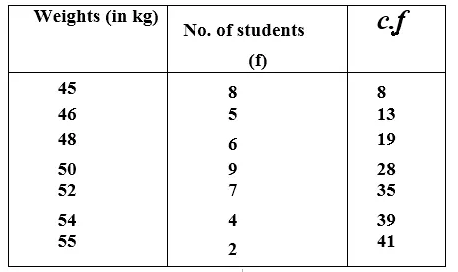Here, number of terms (n) = 41, which is odd,
Median = $$\frac { 41+1 }{ 2 }$$ th term = $$\frac { 42 }{ 2 }$$ th term = 21st term = 50 (∵ 20th to 28th term = 50)
Hence median weight = 50 kg Ans.

Question 9.
Solution:
Arranging first in ascending order, we get: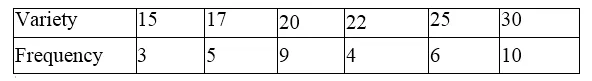Now preparing its cumulative frequency table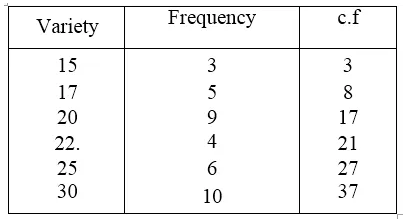Here, number of terms is 37 which is odd.
Median = $$\frac { 37+1 }{ 2 }$$ th term = $$\frac { 38 }{ 2 }$$ th term = 19 th term = 22 (∵18th to 21st = 22)
Hence median – 22 Ans.

Question 10.
Solution:
first arranging in ascending order we get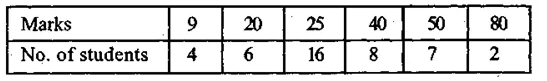Now preparing its cumulative frequency table,we find: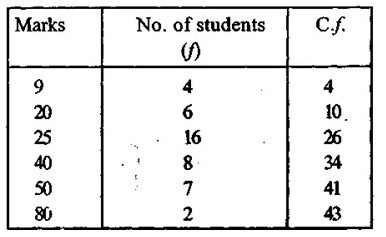Here, number of terms is 43, which if odd.
Median = $$\frac { 43+1 }{ 2 }$$ th term = $$\frac { 44 }{ 2 }$$ th term = 22nd term = 25 25 (∵ 11th to 26th = 25)

Question 11.
Solution:
Arranging in ascending order,we get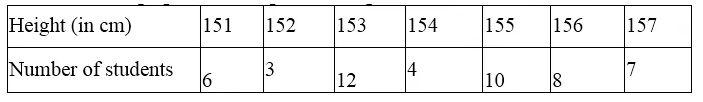Now preparing its cumulative frequency table, we find :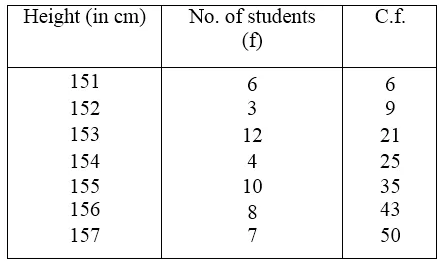Here, number of terms = 50 which is even
∴Median = $$\frac { 1 }{ 2 } \left[ \frac { 50 }{ 2 } th\quad term+\left( \frac { 50 }{ 2 } +1 \right) th\quad term \right]$$
= $$\frac { 1 }{ 2 }$$ (154 + 155) = $$\frac { 1 }{ 2 }$$ (309) = 154.5 (∵ 22nd to 25th = 154, 26th to 34th= 155)

Question 12.
Solution:
Arranging in ascending order, we get: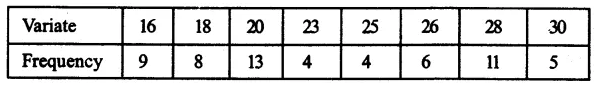Now, preparing its cumulative frequency table.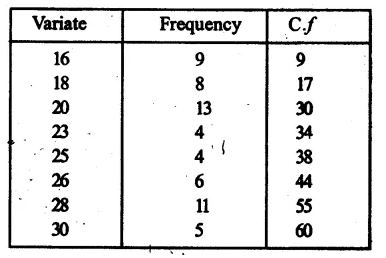Here, number of terms is 60 which is even
∴Median = $$\frac { 1 }{ 2 } \left[ \frac { 60 }{ 2 } th\quad term+\left( \frac { 60 }{ 2 } +1 \right) th\quad term \right]$$
= $$\frac { 1 }{ 2 }$$ (30th term + 31st term)
= $$\frac { 1 }{ 2 }$$ (20 + 23) = $$\frac { 1 }{ 2 }$$ x 43 = 21.5 (∵ 18th to 30th term = 20, 31st term to 34th = 23)
Hence median = 21.5 Ans.

Hope given RS Aggarwal Solutions Class 9 Chapter 14 Statistics Ex 14G are helpful to complete your math homework.

If you have any doubts, please comment below. Learn Insta try to provide online math tutoring for you.

## RS Aggarwal Class 9 Solutions Chapter 14 Statistics Ex 14E

These Solutions are part of RS Aggarwal Solutions Class 9. Here we have given RS Aggarwal Solutions Class 9 Chapter 14 Statistics Ex 14E.

Other Exercises

Question 1.
Solution:
Mean marks of 7 students = 226
∴Total marks of 7 students = 226 x 1 = 1582
Marks obtained by 6 of them are 340, 180, 260, 56, 275 and 307
∴ Sum of marks of these 6 students = 340 + 180 + 260 + 56 + 275 + 307 = 1418
∴ Marks obtained by seventh student = 1582 – 1418 = 164 Ans.

Question 2.
Solution:
Mean weight of 34 students = 46.5 kg.
∴ Total weight of 34 students = 46.5 x 34 kg = 1581 kg
By including the weight of teacher, the mean weight of 35 persons = 500 g + 46.5 kg
= 46.5 + 0.5 = 47.0 kg
∴ Total weight = 47.0 x 35 = 1645 kg
∴ Weight of the teacher = (1645 – 1581) kg = 64 kg Ans.

Question 3.
Solution:
Mean weight of 36 students = 41 kg
∴ Total weight of 36 students = 41 x 36 kg = 1476 kg
After leaving one student, number of students = 36 – 1 =35
Their new mean = 41.0 – 200g = (41.0 – 0.2) kg = 40.8 kg.
∴ Total weight of 35 students = 40.8 x 35 = 1428 kg
∴ Weight of leaving student = (1476 – 1428) kg = 48 kg Ans.

Question 4.
Solution:
Average weight of 39 students = 40 kg
∴ Total weight of 39 students = 40 x 39 = 1560 kg
By admitting of a new student, no. of students = 39 + 1 =40
and new mean = 40 kg – 200 g = 40 kg – 0.2 kg = 39.8 kg
∴Weight of 40 students = 39.8 x 40 kg = 1592 kg
∴Weight of new student = 1592 – 1560 kg = 32 kg Ans.

Question 5.
Solution:
Average salary of 20 workers = Rs. 7650
∴Their total salary = Rs. 7650 x 20 = Rs. 153000
By adding the salary of the manager, their mean salary = Rs. 8200
∴Their total salary = Rs. 8200 x 21 = Rs. 172200
∴Salary of the manager = Rs. 172200 – Rs. 153000 = Rs. 19200 Ans.

Question 6.
Solution:
Average wage of 10 persons = Rs. 9000
Their total wage = Rs. 9000 x 10 = Rs. 90000
Wage of one person among them = Rs. 8100
and wage of new member = Rs. 7200
∴ New total wage = Rs. (90000 – 8100 + 7200) = Rs. 89100
Their new mean wage = Rs.$$\frac { 89100 }{ 10 }$$ = Rs. 8910

Question 7.
Solution:
Mean consumption of petrol for 7 months of a year = 330 litres
Mean consumption of petrol for next 5 months = 270 litres
∴Total consumption for first 7 months = 7 x 330 = 2320 l
and total consumption for next 5 months = 5 x 270 = 1350 l
Total consumption for 7 + 5 = 12 months = 2310 + 1350 = 3660 l
Average consumption = $$\frac { 3660 }{ 12 }$$ = 305 liters per month.

Question 8.
Solution:
Total numbers of numbers = 25
Mean of 15 numbers = 18
∴Total of 15 numbers = 18 x 15 = 270
Mean of remaining 10 numbers = 13
∴Total = 13 x 10 = 130
and total of 25 numbers = 270 + 130 = 400
∴Mean = $$\frac { 400 }{ 25 }$$ = 16 Ans.

Question 9.
Solution:
Mean weight of 60 students = 52.75 kg.
Total weight = 52.75 x 60 = 3165 kg
Mean of 25 out of them = 51 kg.
∴Their total weight = 51 x 25 = 1275 kg
∴ Total weight of remaining 60 – 25 = 35 students = 3165 – 1275 = 1890 kg 1890
Mean weight = $$\frac { 1890 }{ 35 }$$ = 54 kg Ans.

Question 10.
Solution:
Average increase of 10 oarsman = 1.5 kg.
∴ Total increased weight =1.5 x 10 = 15kg
Weight of out going oarsman = 58 kg .
∴ Weight of new oarsman = 58 + 15 = 73 kg Ans.

Question 11.
Solution:
Mean of 8 numbers = 35
∴Total of 8 numbers = 35 x 8 = 280
After excluding one number, mean of 7 numbers = 35 – 3 = 32
Total of 7 numbers = 32 x 7 = 224
Hence excluded number = 280 – 224 = 56 Ans.

Question 12.
Solution:
Mean of 150 items = 60
Total of 150 items = 60 x 150 = 9000
New total = 9000 + 152 + 88 – 52 – 8 = 9000 + 240 – 60 = 9180
∴New mean = $$\frac { 9180 }{ 150 }$$ = 61.2 Ans.

Question 13.
Solution:
Mean of 31 results = 60
∴Total of 31 results = 60 x 31 = 1860
Mean of first 16 results = 58
∴Total of first 16 results = 58 x 16 = 928
and mean of last 16 results = 62
∴Total of last 16 results = 62 x 16 = 992
∴16th result = (928 + 992) – 1860 = 1920 – 1860 = 60 Ans.

Question 14.
Solution:
Mean of 11 numbers = 42
∴Total of 11 numbers = 42 x 11 = 462
Mean of first 6 numbers = 37
∴Total of first 6 numbers = 37 x 6 = 222
Mean of last 6 numbers = 46
∴Total of last 6 numbers = 46 x 6 = 276
∴6th number = (222 + 276) – 462 = 498 – 462 = 36 Ans.

Question 15.
Solution:
Mean weight of 25 students = 52 kg
∴ Total weight of 25 students = 52 x 25 = 1300 kg
Mean weight of first 13 students = 48 kg
∴ Total weight of first 13 students = 48 x 13 kg = 624 kg
Mean of last 13 students = 55 kg
∴Total of last 13 students = 55 x 13 kg = 715 kg
∴Weight of 13th student = (624 + 715) – 1300 = 1339 – 1300 = 39 kg Ans.

Question 16.
Solution:
Mean of 25 observations = 80
Total of 25 observations = 80 x 25 = 2000
Mean of another 55 observations = 60
∴ Total of these 55 observations = 60 x 55 = 3300
Total number of observations = 25 + 55 = 80
and total of 80 numbers = 2000 + 3300 = 5300
Mean of 80 observations = $$\frac { 5300 }{ 80 }$$ = 66.25 Ans.

Question 17.
Solution:
Marks in English = 36
Marks in Hindi = 44
Marks in Mathematics = 75
Marks in Science = x
∴Total number of marks in 4 subjects = 36 + 44 + 75 + x = 155 + x
Average marks in 4 subjects = 50
∴Total marks = 50 x 4 = 200
∴155 + x = 200
=> x = 200 – 155
=> x = 45
Hence, marks in Science = 45 Ans.

Question 18.
Solution:
Mean of monthly salary of 75 workers = Rs. 5680
Total salary of 75 workers = Rs. 5680 x 75 = Rs. 426000
Mean salary of 25 among them = Rs. 5400
Total of 25 workers = Rs. 5400 x 25 = Rs. 135000
Mean salary of 30 among them = Rs. 5700
∴Total of 30 among them = Rs. 5700 x 30 = Rs. 171000
∴ Total salary of 25 + 30 = 55 workers = Rs. 135000 + 171000 = Rs. 306000
∴Total salary of remaining 75 – 55 = 20 workers = Rs. 426000 – 306000 = Rs. 120000
∴ Mean of remaining 20 workers = Rs. $$\frac { 120000 }{ 20 }$$ = Rs. 6000 Ans.

Question 19.
Solution:
Let distance between two places = 60 km
∴Time taken at the speed of 15 km/h = $$\frac { 60 }{ 15 }$$ = 4 hours
and time taken at speed of 10 km/h for coming back = $$\frac { 60 }{ 10 }$$ = 6 hours
Total the taken = 4 + 6 = 10
hours and distance covered = 60 + 60 = 120 km
∴Average speed = $$\frac { 120 }{ 10 }$$ =12 km/hr.

Question 20.
Solution:
No. of total students = 50
No. of boys = 40
∴ No. of girls = 50 – 40 = 10
Average weight of class = 44kg
∴Total weight of 50 students = 44 x 50 = 2200 kg
Average weight of 10 girls = 40 kg
∴Total weight = 40 x 10 = 400 kg
Total weight of 40 boys = 2200 – 400 = 1800 kg
∴Average weight of 40 boys = $$\frac { 1800 }{ 40 }$$kg = 45 kg Ans.

Hope given RS Aggarwal Solutions Class 9 Chapter 14 Statistics Ex 14E are helpful to complete your math homework.

If you have any doubts, please comment below. Learn Insta try to provide online math tutoring for you.